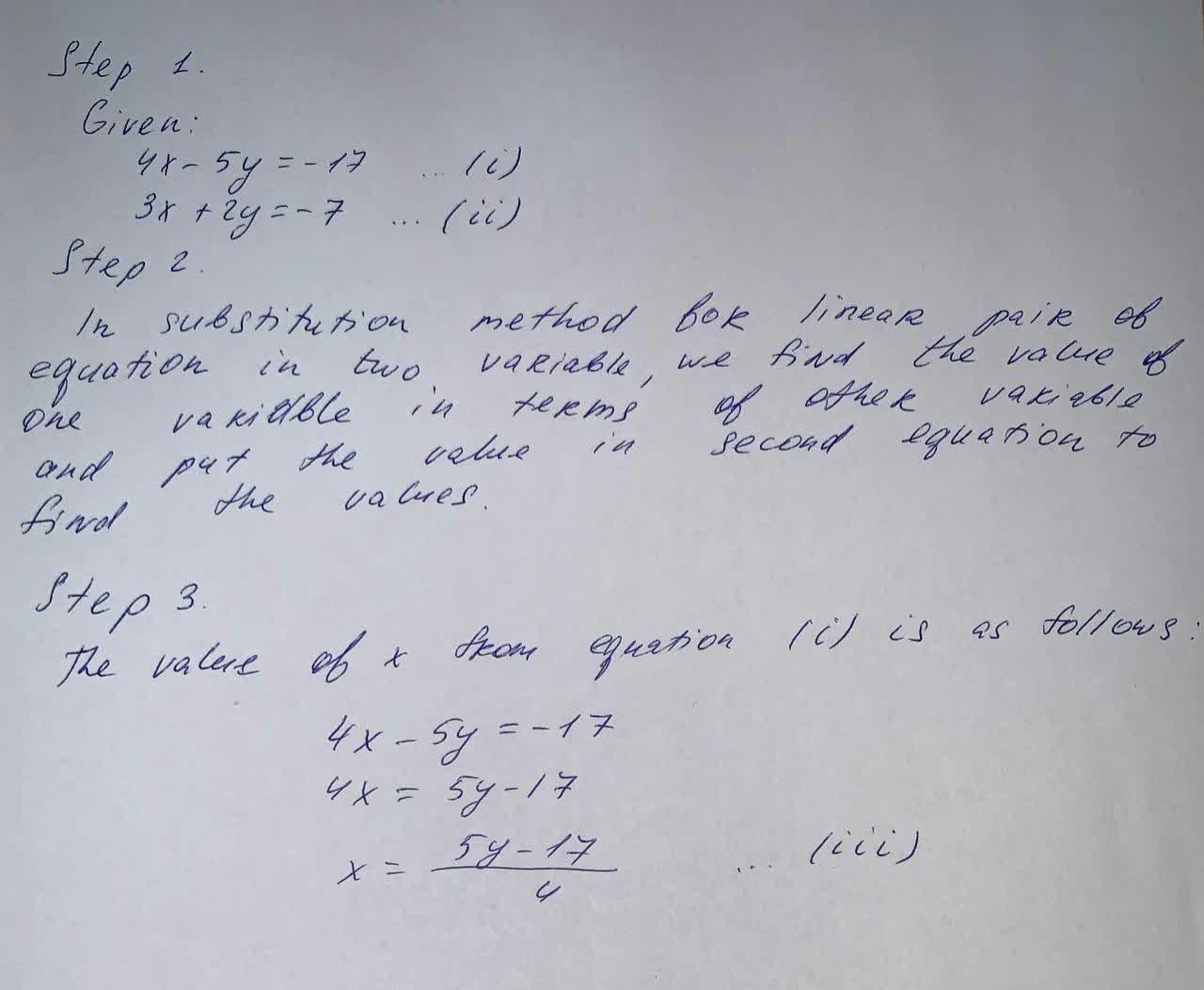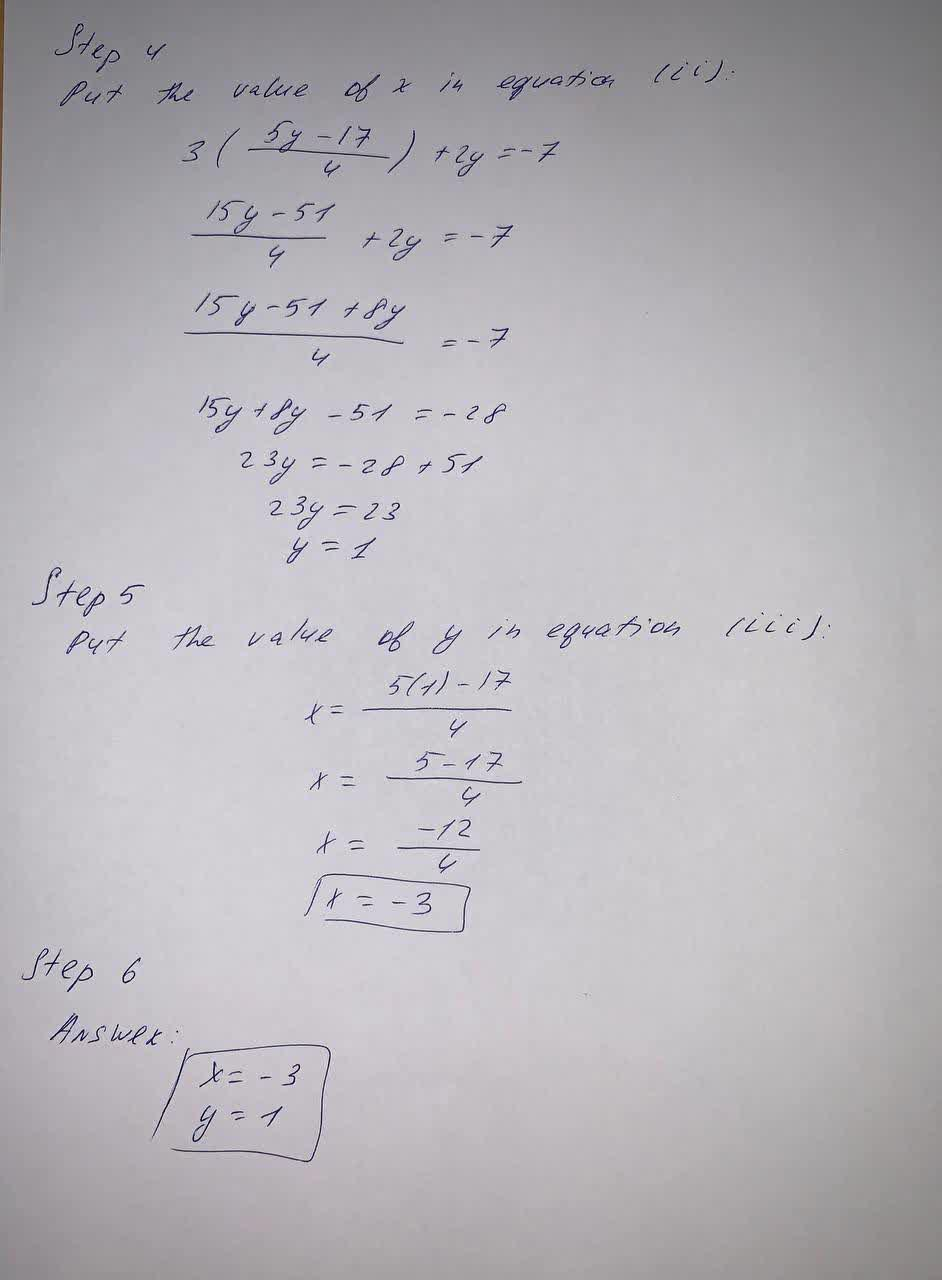# Solve the systems of equations by substitute 4x - 5y = -17, 3x + 2y = -7tabita57i 2021-09-10 Answered

Solve the systems of equations by substitute $\left\{\begin{array}{l}4x-5y=-17\\ 3x+2y=-7\end{array}$

You can still ask an expert for help

• Questions are typically answered in as fast as 30 minutes

Solve your problem for the price of one coffee

• Math expert for every subject
• Pay only if we can solve itNeelam Wainwright